American Journal of Networks and Communications
Volume 4, Issue 6, December 2015, Pages: 119-128

Modeling of Congestion and Traffic Control Techniques in ATM Networks

Bourdilllon Odianonsen Omijeh, Philip Ogah

Department of Electronic & Computer Engineering, University of Port Harcourt, Port Harcourt, Nigeria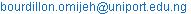(B. O. Omijeh)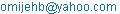(B. O. Omijeh)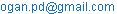(P. Ogah)

Bourdilllon Odianonsen Omijeh, Philip Ogah. Modeling of Congestion and Traffic Control Techniques in ATM Networks. American Journal of Networks and Communications. Vol. 4, No. 6, 2015, pp. 119-128. doi: 10.11648/j.ajnc.20150406.11

Abstract: In this Paper, Computer – based Simulation models for effective Congestion control and Traffic management in Asynchronous Transfer Mode (ATM) network have been developed providing a basis for monitoring ATM networks performance for traffic and congestion control purposes ,providing a system with a reduce short -term congestion in ATM networks, and enhancing a fair operation of networks in spite of the challenges in designing ATM traffic management system to make maximal use of network resources. An IDCC scheme was implemented, applying IDCC methodology to the ATM Network. Using analysis performance, limits were created for robust controlled network behaviour, as dictated by reference values of the desired queue length. By tightly controlling output of the controller, the overall network performance was adjusted and also controlled. A simulation tool, MATLAB/SIMULINK, was used for this purpose. An improvement was observed in the delay performance of ATM networks. The results were obtained by running several simulations and populating a table with the outcome over a number of simulation runs. The effectiveness of the congestion control techniques was tested by analysing the dynamic performance of the model through variation of some parameters. The performance of this model proved to be efficient if applied in the ATM network of today.

Kewords: ATM, IDCC, Traffic, Congestion, Model, Matlab/Simulink

1. Introduction

Congestion is seen as a serious "situation in Communication Networks because too many packets are present in a part of the subnet, performance degrades. Congestion in a network may occur when the load on the network (i.e. the number of packets sent to the network) is greater than the capacity of the network (i.e. the number of packets a network can handle)".Therefore in an actual sense, Congestion occurs when resource demands exceed the capacity. As users come and go, so do the packets they send. "During a session, each host may transmit one or more packet flows to the other host a flow is commonly considered to be a series of closely spaced packets in one direction between a specific pair of hosts" 

The main aim of this paper is to develop an effective congestion Control and traffic management model in ATM Networks.

2. Related Works

"ATM congestion can be defined as a state of network elements in which the network is not able to guarantee the negotiated Network Performance objectives for the already established connections Congestion can be caused by unpredictable statistical fluctuations of traffic flows Since an ATM network supports a large number of bursty traffic sources, statistical multiplexing can be used to gain bandwidth efficiency, allowing more traffic sources to share the bandwidth. But if a large number of traffic sources become active simultaneously, severe network congestion can result" .

"In ATM networks, such circuits are called virtual circuits (VCs) The connections allow the network to guarantee the quality of service by limiting the number of VCs. Typically, a user declares key service requirements at the time of connection set up, declares the traffic parameters and may agree to control these parameters dynamically as demanded by the network Asynchronous Transfer Mode (ATM) networks fulfill high-rate transmissions and heterogeneous requirements with very high rates and different types of services" .

"On the other hand, they are less predictable networks These "types of traffic patterns imposed on ATM networks, as well as the transmission characteristics of those networks, differ markedly from those of other switching networks. One reason is due to the applications that may generate very different traffic patterns and they require different network services. Another reason for this is that trusted statistics from traffic characteristics and connection establishment patterns are not given".

"ATM networks also guarantee the engaged Quality of Service(QoS), so when a connection is accepted, the network must fulfill all the agreements reached with the user. In effect, the network and the subscriber inter into a traffic contract. But due to the statistical multiplexing of the traffic, an inherent feature of ATM, it is possible to have congestion even though all connections carry out their contracts" .

"On the one hand, networks need to serve all user requests for data transmission, which are often unpredictable and bursty with regard to transmission starting time, rate, and size.

On the other hand, any physical resource in the network has a finite capacity, and must be managed for sharing among different transmissions".

2.1. Mathematically Speaking

"Network protocols which use aggressive retransmissions to compensate for packet loss tend to keep systems in a state of network congestion, even after the initial load has been reduced to a level which would not normally have induced network congestion. The stable state with low throughput is known as congestive collapse ".

2.2. Congestion Control

"Congestion control mechanisms are however divided into two categories: one category prevents the congestion from happening (Preventive congestion control) and the other category removes congestion after it has taken place’ (Reactive congestion control).

2.3. Traffic Management

"Monitoring ATM networks performance for traffic and congestion control purposes leads to difficulty because there are some parameters whose values must be tuned. These parameters are not only based on users’ traffic descriptors but on the different traffic control policies implemented".

In ATM network, traffic policing is located at the access point to the User Network Interface (UNI). What traffic policing does is to check the validity of VPI and VCI (Virtual Channel Identifier) values and monitor the cells arriving at the network and determines whether it violates any parameters. In ATM network, two most popular methods exist to control the peak rate, mean rate and different load States. These are the Leaky Bucket method and Window Method otherwise referred to as the Usage parameter Control (UPC) according to Uyles Black (1995). The UPC detects any nonconforming sources and the necessary actions it takes are as follows:

i.        To dropping violating cell

ii.        To delay violating cells in queue

iii.        To mark violating cells and treat them accordingly

iv.        To control traffic by informing the source to take action when violation occurs.

"One of the earliest documents that mention the term ‘congestion collapse’ is by John Nagle; here, it is described as a stable condition of degraded performance that stems from unnecessary packet retransmissions. Nowadays, it is, however, more common to refer to ‘congestion collapse’ when a condition occurs where increasing sender rates reduces the total throughput of a network" ."The existence of such a condition was already acknowledged in  which even uses the word ‘collapse’ once to describe the behaviour of a throughput curve". "Therefore, even if a network adopts a strategy of congestion avoidance, congestion recovery schemes would still be required to retain throughput in the case of abrupt changes in a network that may cause congestion" .

"Congestion control mechanisms are divided into two categories, namely: Open Loop and Closed Loop. In this open loop, policies are used to prevent the congestion before it happens. Congestio control is handled either by the source or by the destination while in the closed loop the congestion is removed after it happens" 

2.4. Traffic Modeling

A key element in simulating or analyzing communication networks is traffic modeling. "Traffic models reflect our best knowledge of traffic behavior" . "Traffic is easier to characterize at sources than within the network because flows of traffic mix together randomly within the network. When flows contend for limited bandwidth and buffer space, their interactions can be complex to model"[12-14].

In order to obtain a successful performance evaluation, a clear understanding of the following is crucial: (i) The selection of a suitable random traffic model and (ii) The pattern or nature of the traffic in the target system.

3. Simulated Design of a Congestion and Traffic Management Model

The Design Stages involved in the work include: (i) Developing a dynamic Fluid Flow Model: This has the ability to capture the essential dynamics of the system. (ii) Designing of inflow to the switch: This is helps in regulating the flow of traffic at the source (consumer/user end) into the ATM network (iii) Designing of a simple non-linear congestion controller: This model attempts to keep a queue buffer length close to a reference value without knowledge or measurement of the flow in for both available (best effort) and guaranteed sources. (iv) Design of the queuing state model in the buffer of our ATM design.(v) Designing of Linear Controller Model Subsystem: For the implementation of congestion control by an Integrated dynamic congestion controller (IDCC).

3.1. Congestion Control in an ATM Network

The block diagram of the congestion control in an ATM Network is represented in Figure 1.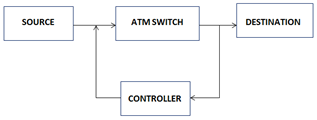Figure 1. Block Diagram of Congestion Control System.

Source: This originates the traffic that transverses the links and ATM switches of an ATM network. This traffic which could be in form of data, voice or video. ABR traffic source (which is a model of real time services) is represented as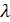ABR (t).

ATM Switch: This "accepts the incoming cell from an ATM source or another ATM switch. It then reads and updates the cell-header information and switches the cell to an output interface toward its destination. The basic operation of an ATM switch is easy: The cell is received across a link on a known VCI (Virtual channel identifier) or VPI (Virtual path identifier) value". For a dynamic fluid flow model of ABR "traffic through the buffer of an ATM switch and assuming the link has a First-In-First-Out (FIFO) service discipline, the following standard assumptions are made: packets arrive according to a Poisson process, packet transmission time is proportional to packet length, and that the packets are exponentially distributed with mean length 1".

The queue state model is given in (1)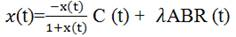(1)

For several numbers of switches (1) can further be extended to (2).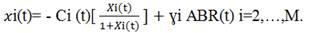(2)

Where

C i(t) = bandwidth (capacity, cell service rate)allocated to the ABR traffic at node i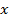i(t) = state of the queue (i.e. ensemble average of number of cells in the queue) at node i,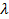ABR (t) = arrival rate due to ABR traffic

ɣi ABR(t)= ABR traffic entering node i, arriving from the previous node, delayed by a deterministic amount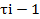due to the transmission propagation and is further represented in Equation(3)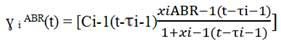(3)

WhereI ABR (t) is the ensemble average of the number of cell places in the buffer occupied by ABR traffic.

3.2. Integrated Dynamic Congestion Controller

The controller used in the work is the IDCC (Integrated Dynamic Congestion Controller) as shown in Figure 2.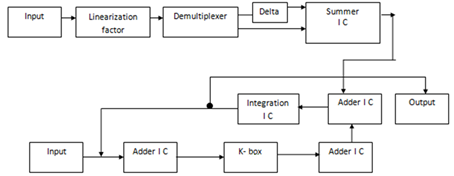Figure 2. Block Diagram of the IDCC Architecture.

Input: The controller input is usually a control signal denoted by (4).

Input1 = C max - Cp(t)                           (4)

Where, C max = maximum rate at which packets is transmitted

Cp(t) = control signal determined by the congestion controller.

Linearization factor (Pi(x)): This helps to simplify our design procedure and reduce the model to a linear one as shown in (5)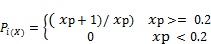(5)

Where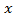p = state of the queue or queue length.

Demultiplexer: This actually helps to separate combined signal into separate single signal component.

Delta (∆): This denotes model uncertainties.

Summer IC: This circuitry is similar to the multiplexer, it tends to add two different signals together.

K-box: This box is comprised of what is known as the controller factor (K) as shown in (6)

Where

K= Kc * Kc_bar                            (6)

Kc = x/(x +1)                            (7)

Integration IC: This circuitry is responsible for producing outputs that are integral of its input at the current time step. The following equation represents the output of the block y as a function of its input u and an initial condition y0, where y and u are vector functions of the current simulation time t.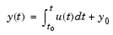The selected control strategy for the switch is developed using (2) as follows:

Let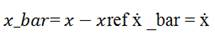(8)

Then from Equation (3.2), we have: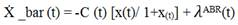(9)

The control input is given by (10) below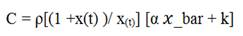(10)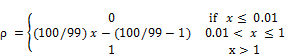(11)

K_dot  = Pr[δ x bar]                     0≤k≤Ǩ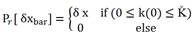(12)

A, δ > 0 and Ǩ >=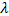ABR (t) are design constants.

Ẋ_bar (t) =- ρ δ x – ρ k o+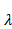1ABR

Where k o =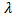1ABR Ǩ = k + k o and k d = Pr[δ x_ bar]

For this research work, the following Lyapunov’s function for analysis was proposed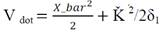The time derivative of V from (10) and (11) is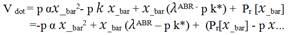(13)

Equation (13) can also be write as,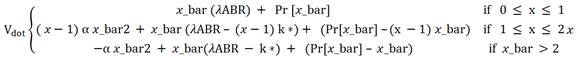(14)

Examining the properties of V dot we have

V dot = 0 for_bar = 0, and V dot< 0 for_bar ≠ 0

From Lyapunov theory and additional arguments it can be deduced that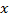_bar is bounded and_bar (t) → 0 as t → ∞ .With the above choice of C we can confidentially state that the maximum possible value C max of C satisfies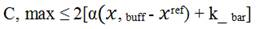(15)

Where α and k _bar are design constants which provide some flexibility in meeting possible constraints.

3.3. Algorithm for Computing Process in a Congestion Controller

From (2), we have that the control strategy of our IDCC controller is given by,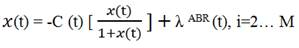The control input described in (3) is given by,

C= ρ [(1 +x (t))/ x (t)] [α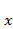_bar + k]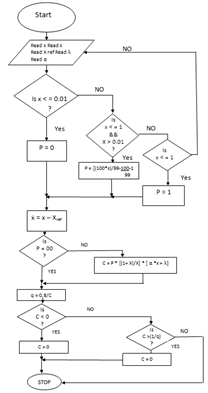Figure 3. Algorithm for computing process in congestion controller.

From Figure3 q= cell service time and x (t), α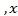_bar are kept constant.

The meanings of the symbols are treated in (2).The flow process is shown in Figure 4.

3.3.1. Computation Process of the Controller

This process is shown in Figure 3.

The process of setting the reference value for an available traffic is elucidated in Fig 4 below.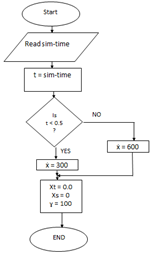Figure 4. A flowchart of reference value setting in Available traffic.

Where, sim-time = simulation time

Xt = total queue length

Xs = Reading at start time

ɣ = ABR traffic entering a switching node

3.3.2. Setting Reference Value for Abr Traffic

DESTINATION: This serves as the final end point for the traffic that transverses the links and ATM switches of an ATM network.

3.4. Modeling of Dynamic Fluid Flow Model and Non-Linear Congestion Controller

The Matlab model of congestion control in ATM network is better understood by representing a dynamic fluid flow model on Matlab/simulink as shown in Figure 5 developed in this work.

3.4.1. Dynamic Fluid Flow Model

This model is made up of the source(s), a flow controlled subsystem, transmission delay, a switch and non-linear controller subsystem and the destination.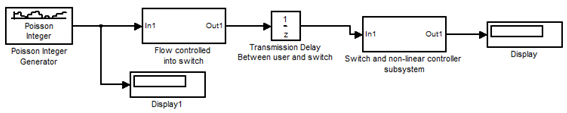Figure 5. Computer-based Design of Dynamic Fluid Flow Model.

3.4.2. Poisson Integer Generator

The Poisson Integer Generator block models the ABR traffic that transverses through the ATM network.it generates random integers using a Poisson distribution.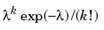(16)

where; λ = positive number known as the Poisson parameter, exp = base of the natural logarithm.

3.4.3. Non-Linear Congestion Controller

The model as designed in this work is seen in Figure 6.

3.4.4. Transmission Delay

This deals with delay in transmission link between the ABR traffic source generator(consumer) and ATM switch. The Unit Delay block delays controlled ABR traffic by sample period of -1 seconds. The delay block is used to model transmission delay and it is Equivalent to the z-1 discrete-time operator. The block accepts the ABR traffic input and generates one output. If the input is a vector, all of its elements are delayed by the same sample period.

The value of ABR traffic due to delay is stated in (2).

3.4.5. Switch and Non-Linear Controller Subsystem

This subsystem model and its derivative are given in (1) (8) (9) & (14).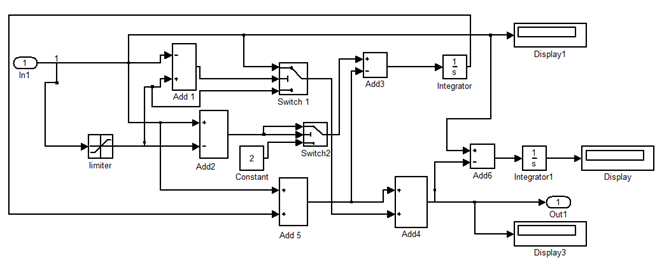Figure 6. Non-Linear Congestion Controller Model.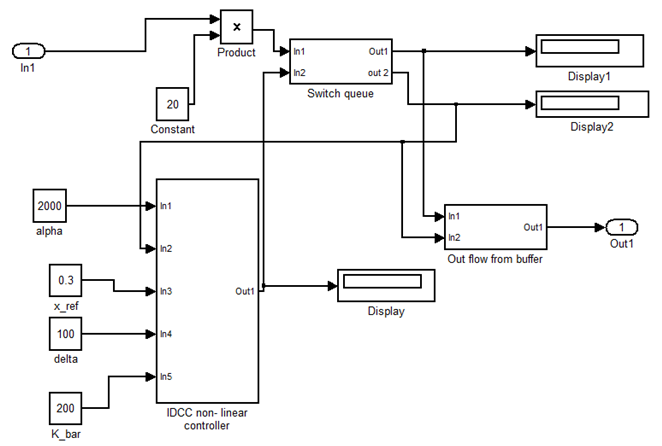Figure 7. Designed Switch and Non-Linear Controller Subsystem.

This subsystem is divided into:

i. ATM Switch subsystem

ii. IDCC (Integrated dynamic congestion controller) subsystem

iii. Out flow from buffer subsystem.

3.4.6. ATM Switch Subsystem

The model for this ATM switch is given in (1).

The ATM switch subsystem as seen in Fig 3.9, is made up of various systems/ blocks that work together, according to Equation(1), inorder to achieve its switching function. The Cserver (finite buffer) is the bandwidth (capacity, cell service rate) allocated to the ABR traffic at the ATM switch and xbuff is the maximum possible queue size for the ABR traffic. The relationship between Cmax, α,buff,ref, k_bar is given and defined in (14).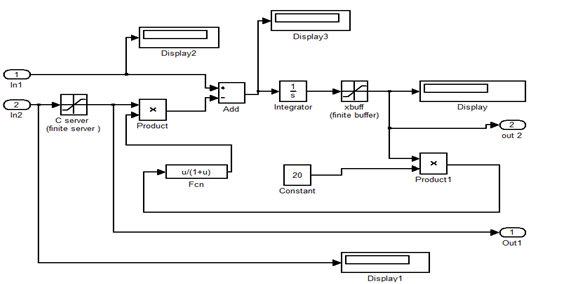Figure 8. ATM Switch Subsystem Model.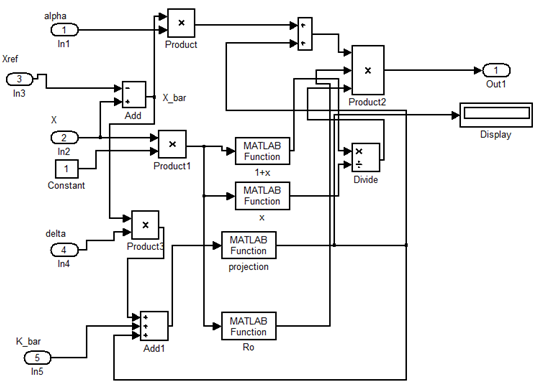Figure 9. IDCC Model.

3.4.7. IDCC (Integrated Dynamic Congestion Controller) Subsystem

The IDCC controller is based on the algorithm shown in Equation (4) below.

It is made up of five (5) input namely: ẍ, xref , α , δ and ǩ and one output (C) that is fed back into the ATM switch.

a.   ẍ (represented by X_bar in Fig 3.10) is given by the difference between X and Xref as stated in (8).

b.   Xref is a constant set by the designer or network operator for adjusting the ATM switch queue state.

c.   α, δ and ǩ (represented by alpha, delta and K_bar in Figure 9) are the design constants for the non-linear controller.

The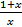part of (10) is represented by the MATLAB function in Figure 8 and ρ represented by a MATLAB function Ro in Figure 9.

3.4.8. Out Flow from Buffer

Table 1. ABR sources.

 Parameter Values Specification Lambda 10 Poisson generator Initial seed 43 Poisson generator Sample time 0.005 Poisson generator

This MATLAB model in Figure 10 represents the outflow of the ATM switch to the next node which can be either a destination or another ATM switch.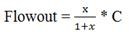(17)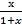= MATLAB Fcn in Fig .10 above

C = the output of cell service rate.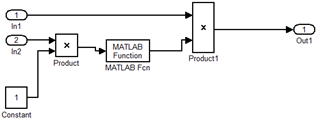Figure 10. BUFFER Model.

Parameters adopted for this simulation are stated in Table 1, 2, 3 and 4.

Table 2. Switch queue.

 Parameter Values Specification Upper limit 112040 C server Lower limit 0 C server Initial condition 0 Integrator Upper limit 128 Xbuff Lower limit -128 Xbuff Expression u/(1 + u) Fcn

Table 3. ATM/flow controlled into switch.

 Parameter Values Specification upper limit 2 limiter Lower limit -0.5 limiter Threshold 0 switch1 Threshold 0 switch2 initial condition 0 Integrator initial condition 0 Integrator1

Table 4. IDCC.

 Parameter Values Specification alpha(α) 2000 constant Xref 0.2 constant delta(δ) 100 constant K_bar 200 constant

4. Results and Discussion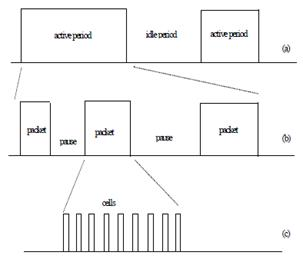Figure 11. Display of packets and Cell placement for calculation purpose.Figure 12. Simulation Result.

In this research work we evaluate the performance properties of the proposed strategy using a 1-node network model, The xmt_ws source is a Poisson distribution source modelled as shown in Figure 4, with a lambda () of 10. The sample "period between packets is 0.0005 seconds. The ATM switch has a finite output buffer (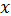) of 128 cell places with a reference point(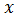ref) set to 0.2 cell places. "The controller design variable α is selected equal to 10000, and the ISS Filter period (for calculating new Service Rate) is set at 112040 cell times".

Both model in (1); and "MATLAB simulation consider the same input representing a bursty on-off source, shown in Figures 11&12 (MATLAB output). The active and idle periods of the connection are shown in part (a), the packet activity in part (b), and the cell activity in part (c) of Figure 11. The idle period has a geometric distribution with the mean value chosen to adjust the network load. During each active period a number of packets are generated with a geometric distribution and mean number of packets N".

The time evolution of the queue state from MATLAB simulation is presented in Figure 12. From Figure 12, we can observe that there is a reasonable agreement between the proposed model and the required one as described in (1) .

The simulations of the simple model shown in Figure 4. together with the controller in Figure 8 using MATLAB demonstrate the "validity of the model and robustness of the proposed controller with respect to model uncertainties and noise effects".

IDCC (Integrated Dynamic Congestion Controller):

We test a variety of cases in order to allow us to evaluate the responsiveness and robustness of our control design. The results generated when the reference point is set to 0.2, 0.3 and 0.4 are represented below in Table 1, 2 and 3 respectively. For example at t = 0.10 sec the ABR queue is 0.3660 cells. At t=0.50 we want ABR queue to be dropped down to 0.4318 cells. This means that we can either increase the switch service rate available for ABR traffic or regulate at the ABR sources to send less traffic. Also notice that in Table .2 and 3, there was a drop a the same time. Things get more complicated when real-time services get more demanding. This means that the ABR sources must again drop its sending rate. At t=0.5 ABR sources have more bandwidth represented by C (t) in Table 1, 2 and 3. It shows that the controller adapts very quickly to the set reference point. Also a graph of C(t) ( which is the bandwidth (capacity, cell service rate) allocated to the ABR traffic at the switch) and X(t)(which is the state of the queue (i.e. ensemble average of number of cells in the queue) at reference point of 0.2,0.3 and 0.4 against the MATLAB simulation time.

Table 5. Xref = 0.2.

 time X(t) C(t) Xbar = X(t) - Xref 0.1 0.366 154.9 0.266 0.2 0.3729 173.4 0.1729 0.3 0.4035 258.1 0.2035 0.4 0.4474 385.9 0.2474 0.5 0.4318 339.5 0.2318 0.6 0.4309 337 0.2309 0.7 0.3758 181.3 0.1758 0.8 0.4144 289.1 0.2144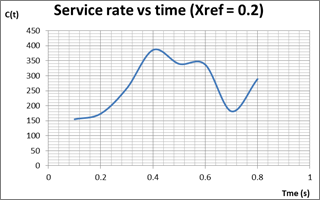Fig. 13a. Service Rate vs Time (Xref=0.2).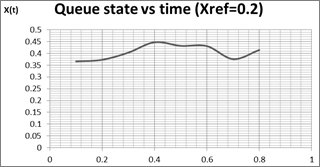Fig. 13b. Queue state vs time (Xref=0.2).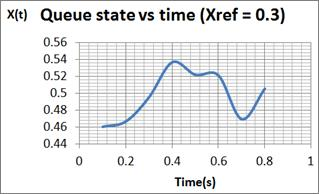Fig. 14a. Queue state vs time (Xref=0.3).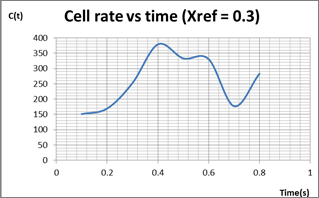Fig. 14b. Cell rate vs time ( Xref=0.3).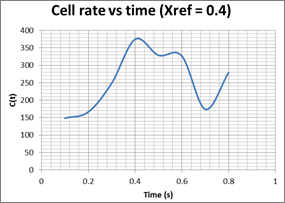Fig. 15a. Cell rate vs time (Xref=0.4).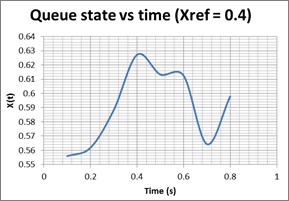Fig. 15b. Queue state vs time ( Xref=0.4).

5. Conclusion

A number of simulations were carried out using a computer – based approach and the system was evaluated. The poisson generator model was useful in, reproducing the phenomena in Fig 4.3 of ABR (Available Bit Rate) traffic. A number of network models were developed and results were obtained for the modelled systems.

The proposed scheme for congestion control uses the IDCC approach. A specific challenging formulation for managing traffic, passing through the output port of the switch is represented. IDCC is developed from non-linear control theory using a fluid flow model. This fluid flow model represents the dynamical system behavior, taking advantage of the packet flow conservation considerations and by matching the queue behavior at equi-state. In this way analytical performance limits can be abstracted, for a robust controlled network behaviour. We focused on ABR traffic service (which is a type of real time service). A supplementing study can be carried out to further represent other traffic services and also assess the performance of the system.

References

1. Dinesh Taukur: What is Congestion,.www.ecomputernote.com
2. ROBERTS, J 2004, Internet Traffic, Qos, And Pricing. Proceedings Of The IEEE, 92, 1389-1399.
3. TORSTEN BRAUN & THOMAS STAUB 2008, End-To-End Quality Of Service Over Heterogeneous Networks. Springer. ISBN 978-3-540-79119-5.
4. WILLIAM STALLINGS, "High Speed Networks TCP/IP And ATM Design Principles", Prentice Hall,1998.
5. LEONARD FRANKEN. Quality Of Service Management: A Model-Based Approach. Phd Thesis, Centre For Telematics And Information Technology, 1996.
6. JACOBSON V 1998, Notes On Using Red For Queue Management And Congestion Avoidance, Viewgraphs From Talk At Nanog 13, Available From Ftp://Ftp.Ee.Lbl.Gov/Talks/Vj-Nanog-Red.Pdf.
7. NAGLE J 1984, Congestion Control In IP/TCP Internetworks RFC 896.Networking, June 1993.
8. GERLA M AND KLEINROCK L (1980) Flow Control: A Comparative Survey. IEEE Transactions On Communications 28 (4), 553–574.
9. CHARNY, A. (1994). "An Algorithm For Rate Allocation In A Cell-Switching Network With Conference Paper At Adekunle Ajasin University, Akungba – Akoko, 2010.
10. KESHAV, S 1991B, A Control-Theoretic Approach To Flow Control ACM SIGCOMM, Pp. 3–15. ACM Press.
11. Omijeh.B.O and Biebuma.J.J(2013). Traffic Modelling For Capacity Analysis of CDMA Networks using Lognormal Approximation Method, IOSR Journal of Electronics and Communication Engineering (IOSR-JECE),Volume 4, Issue 6 (Jan. - Feb. 2013), PP 42-50.
12. THOMAS, M & CHEN 2007, Network Traffic Modeling, The Handbook Of Computer Networks, Hossein Bidgoli (Ed.), Wiley, To Appear 2007.Southern Methodist University, Dallas, Texas.
13. AL-BAHADILI, H. 2012: Simulation In Computer Network Design And Modeling, Use And Analysis. Hershey, PA, IGI Global.
14. Omijeh, B.O, R. Okinege, R.Ochi (2013): "Traffic Modelling for capacity analysis of CDMA Networks using Gaussian, International Journal of Electronic , Communication and Computer Engineering (IJECCE), Vol 5, N0. 3, 2013.
15. http://www.eng.auburn.edu
16. http://www.cs.ucy.ac.cy
17. Network Congestion :http:// en.wikipedia.org/wiki/network_congestion
18. ARANUWA, F,O 2010, Traffic Control And Bandwidth Allocation In ATM Networks.
19. Welze (2006): Network congestion Control: Managing Internet Traffic, www.onlinelibrary.wiley.com

 Contents 1. 2. 2.1. 2.2. 2.3. 2.4. 3. 3.1. 3.2. 3.3. 3.4. 4. 5.
Article ToolsAbstractPDF(2621K)